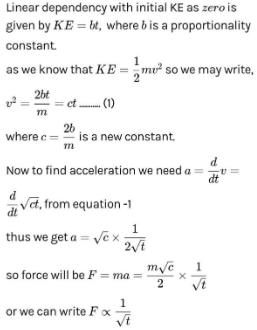Courses

# Test: NEET Previous Year Questions: Work, Energy & Power- 1

## 22 Questions MCQ Test Physics Class 11 | Test: NEET Previous Year Questions: Work, Energy & Power- 1

Description
This mock test of Test: NEET Previous Year Questions: Work, Energy & Power- 1 for JEE helps you for every JEE entrance exam. This contains 22 Multiple Choice Questions for JEE Test: NEET Previous Year Questions: Work, Energy & Power- 1 (mcq) to study with solutions a complete question bank. The solved questions answers in this Test: NEET Previous Year Questions: Work, Energy & Power- 1 quiz give you a good mix of easy questions and tough questions. JEE students definitely take this Test: NEET Previous Year Questions: Work, Energy & Power- 1 exercise for a better result in the exam. You can find other Test: NEET Previous Year Questions: Work, Energy & Power- 1 extra questions, long questions & short questions for JEE on EduRev as well by searching above.
QUESTION: 1

### The minimum velocity (in ms-1) with which a car driver must traverse a flat curve of radius 150 m and coefficient of friction 0.6 to avoid skidding is  [AIEEE 2002]

Solution:

To move a body on a circular path, centripetal force is necessary. In this question, there is an agent, friction that will provide the required centripetal force to the car.
Using the relation,
mv2r=μR,R=mg
mv2r=μmg
or, v2=μrg
or, v2=0.6×150×10
or, v=30m/s

QUESTION: 2

### Which of the following statements is false for a particle moving in a circle with a constant angular speed ? [AIEEE 2004]

Solution:

In uniform circular motion tangential acceleration is zero only centripetal acceleration work which is directed towards centre.

QUESTION: 3

### An angular ring with inner and outer radii R1 and R2 is rolling without slipping with a uniform angular speed. The ratio of the forces experienced by the two particles situated on the inner and outer parts of the ring, F1/F2 is

Solution:

F = mω2R
Since F α R, the ratio of forces will be equal to the ratio of radii.
Hence D is the correct answer.

QUESTION: 4

A point P moves in counter-clockwise direction on a circular path as shown in the figure. The movement of P is such that it sweeps out a length s = t3 + 5, where s is in metre and t is in second. The radius of the path is 20 m. The acceleration of P when t = 2s is nearly

[AIEEE 2010]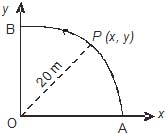Solution:

It is given that s = t3 + 5
Thus we get v =  3t
And a = 6t
Thus acceleration when t = 2 is, a = 12m/s2

QUESTION: 5

For a particle in uniform circular motion the acceleration at a point P(R,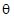) on the circle of radius R is (here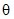is measured from the x-axis)

[AIEEE 2010]

Solution: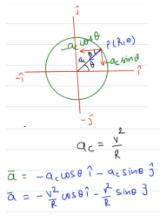QUESTION: 6

In a typical power station, amid a series of energy transfers and conversions, about 70% of energy input gets wasted in form of thermal energy, efficiency of such a power station is about

Solution:

Assume total input energy to be 100%
Lost energy =70%
Left energy = 100 – 70 = 30 % is the output energy
Efficiency = output energy/input energy = 30/100 = 0.3
Percentage efficiency = 0.3 × 100 = 30%

QUESTION: 7

A spring of force constant 800 N/m has an extension of 5 cm. The work done in extending it from 5 cm to 15 cm is

[AIEEE 2002]

Solution:

The work done is stored as elastic potential energy in the spring. It is given by
W = 1/2 * k (x₂2 - x₁2
x₁ = 5 cm
x₂ = 15 cm
Force constant, k = 800 N/m = 800/100 N/cm = 8 N/cm
W = 1/2 * 8 (15^2 - 5^2) = 1/2 * 8 (225 - 25)
W = 1/2 *  8 * 200 = 800 N cm
W = 800 N cm = 8 N m = 8 J
Therefore the work done in extending it from 5cm to 15cm is 8 J.
Hence B is the correct answer.

QUESTION: 8

A body is moved along a straight line by a machine delivering a constant power. The distance moved by the body in time t is proportional to

[AIEEE 2003]

Solution:

P= fv
P = mav
p = mdv/dt.v
p.dt/m = v.dv ( integration from 0-t)
pt/m = v²/2
v = √(2pt/m)
ds/dt = t½dt
s = t3/2

QUESTION: 9

A spring of spring constant 5 × 103 N/m is stretched initally by 5 cm from the unstreched position. Then the work required to stretch it further by another 5 cm is

[AIEEE 2003]

Solution:

work done in stretching a spring by a distance x is
W=½​kx2
where k is the spring constant.
work required to stretch the string by 10cm is
W1​=½​kx2=½ ​5×103(0.1)2=25J
work required to stretch the string by 5cm is
W2​=½​kx2=½ ​5×103(0.05)2=6.25J
Hence the work done in stretching it from 5cm to 10cm is
W1​−W2​=25−6.25=18.75J

QUESTION: 10

A force F = (5i + 3j +2 k) N is applied over a particle which displaces it from its origin to the point r = (2 i - j) m. The work done on the particle in joule is

[AIEEE 2004]

Solution: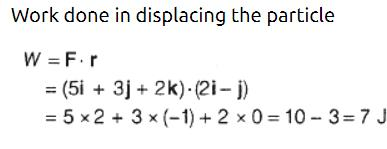QUESTION: 11

A uniform chain of length 2 m is kept on a table such that a length of 60 cm hangs freely from the edge of the table. The total mass of the chain is 4 kg. What is the work done in pulling the entire chain on the table?

[AIEEE 2004]

Solution:

The center of mass of the hanging part is at 0.3m from table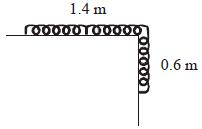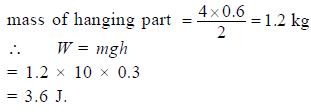QUESTION: 12

A particle moves in a straight line with retardation proportional to its displacement. Its loss of kinetic energy for any displacement x is proportional to

[AIEEE 2004]

Solution:
QUESTION: 13

The block of mass M moving on the frictionless horizontal surface collides with the spring of spring constant k and compresses it by length L. The maximum momentum of the block after collision is

[AIEEE 2005]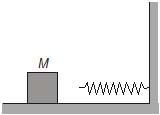Solution: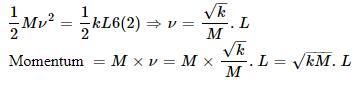QUESTION: 14

A spherical ball of mass 20 kg is stationary at the top of a hill of height 100 m. It rools down a smooth surface to the ground, then climbs up another hill of height 30 m and finally rolls down to a horizontal base at a height of 20 m above the ground. The velocity attained by the ball i

[AIEEE 2005]

Solution:

mgh = 1/2×mv2
v = √2gh
v = √2×10×80(actually 80 is come out of from 100-20)
v = 40 m/s
Hence A is correct.

QUESTION: 15

A body of mass m is accelerated uniformly from rest to a speed V in a time T. The instantaneous power delivered to the body as function of time, is given by

[AIEEE 2005]

Solution: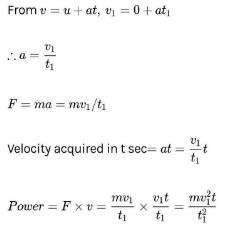QUESTION: 16

A bullet fired into a fixed target loses half of its velocity after penetrating 3 cm. How much further it will penetrate before coming to rest, assuming that it faces constant resistance to motion ?

[AIEEE 2005]

Solution: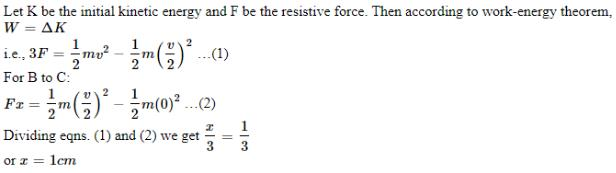QUESTION: 17

A mass of M kg is suspended by a weightless string. The horizontal force that is required to displace it until the string makes an angle of 45° with the initial vertical direction is

[AIEEE 2006]

Solution:

By the Work Energy Theorem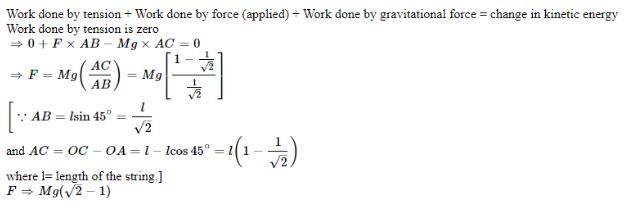QUESTION: 18

A ball of mass 0.2 kg is thrown vertically upwards by applying a force by hand. If the hand moves 0.2 m while applying the force and the ball goes upto 2 m height further, find the magnitude of the force. Consider g = 10 m/s

[AIEEE 2006]

Solution: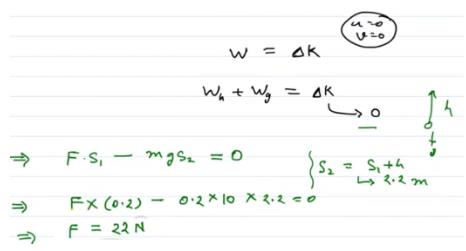QUESTION: 19

The potential energy of a 1 kg particle free to move along the x-axis is given by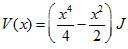The total mechanical energy of the particle is 2 J. Then, the maximum speed (in ms_1) is

[AIEEE 2006]

Solution: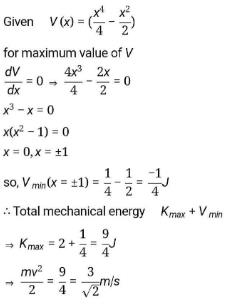QUESTION: 20

A 2 kg block slides on a horizontal floor with a speed of 4 m/s. It strikes a uncompressed spring, and compresses it till the block is motionless. The kinetic friction force is 15 N and spring constant is 10000 N/m. The spring compresses by

[AIEEE 2007]

Solution: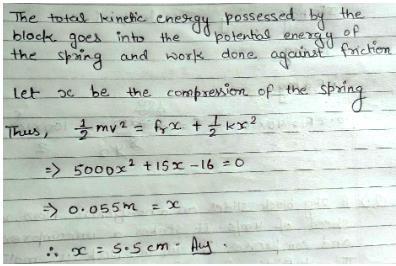QUESTION: 21

An athlete in the olympic games covers a distance of 100 m in 10 s. His kinetic energy can be estimated to be in the range

[AIEEE 2008]

Solution: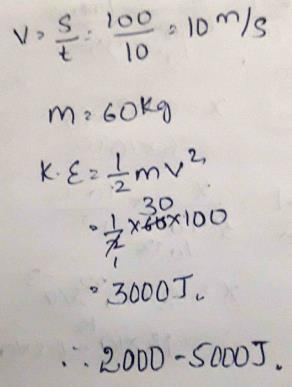QUESTION: 22

At time t = 0 s particle starts moving along the x-axis. If its kinetic energy increase uniformly with time t, the net force acting on it must be proportional to

[AIEEE 2011]

Solution: